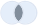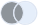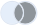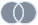# About data joins

You can create a dataset from data sources (tables) that are related by one or more common columns. To create a dataset, you need to combine these tables based on their common columns. The method used for combining the tables is called data joining. The result of the join is a virtual table that contains data from the tables that are parts of the join.

A join can be based on one or more common columns.

There are four join types:

•Inner join
•Left join
•Right join
•Full outer join

## Inner join

When using an inner join to combine two tables, the result is a table that contains only the rows with values in both tables.

## Left join

When using a left join to combine two tables, the result is a table that contains all the rows from the left table and corresponding values from the right table. If a row from the left table does not have values in the right table, then null values appear in the resulting table.

## Right join

When using the right join to combine two tables, the result is a table that contains all the rows from the right table and corresponding values from the left table. If a row from the right table does not have values in the left table, then null values appear in the resulting table.

## Full outer join

When using a full outer join to combine two tables, the result is a table that contains all rows from both tables. If a row from any of the tables does not have values in the other table, then null values appear in the resulting table.